# Bar Charts

library("lessR")
##
## lessR 3.9.8  feedback: gerbing@pdx.edu  web: lessRstats.com/new
## ---------------------------------------------------------------
## > d <- Read("")   Read text, Excel, SPSS, SAS, or R data file
##   d is default data frame, data= in analysis routines optional
##
## Access many vignettes to show by example how to use lessR.
## To learn about reading, writing, & manipulating data, graphics,
##   means & models, factor analysis, & customization:
## enter,  browseVignettes("lessR")  or
## visit,  https://CRAN.R-project.org/package=lessR

## Data

Most of the examples are an analysis of data in the Employee data set, included with lessR. First read the Employee data into the data frame d. See the Read and Write vignette for more details.

d <- Read("Employee")
##
## >>> Suggestions
## Details about your data, Enter:  details()  for d, or  details(name)
##
## Data Types
## ------------------------------------------------------------
## character: Non-numeric data values
## integer: Numeric data values, integers only
## double: Numeric data values with decimal digits
## ------------------------------------------------------------
##
##     Variable                  Missing  Unique
##         Name     Type  Values  Values  Values   First and last values
## ------------------------------------------------------------------------------------------
##  1     Years   integer     36       1      16   7  NA  15 ... 1  2  10
##  2    Gender character     37       0       2   M  M  M ... F  F  M
##  3      Dept character     36       1       5   ADMN  SALE  SALE ... MKTG  SALE  FINC
##  4    Salary    double     37       0      37   53788.26  94494.58 ... 56508.32  57562.36
##  5    JobSat character     35       2       3   med  low  low ... high  low  high
##  6      Plan   integer     37       0       3   1  1  3 ... 2  2  1
##  7       Pre   integer     37       0      27   82  62  96 ... 83  59  80
##  8      Post   integer     37       0      22   92  74  97 ... 90  71  87
## ------------------------------------------------------------------------------------------

## Bar Chart of One Variable

One of the most frequently encountered visualizations is the bar chart, created for a categorical variable.

Bar chart: Plot a number associated with each category of a categorical variable as the height of the corresponding bars.

A call to a function to create a bar chart contains the name of the variable that contains the categories to be plotted. With the BarChart() function, that variable name is the first argument passed to the function. In this example, the only argument passed to the function is the variable name as the data frame is named d, the default value. The following illustrates the call to BarChart() with a categorical variable named $$x$$.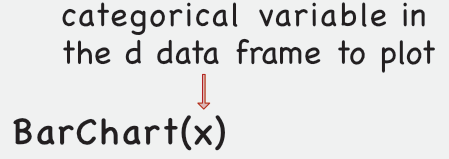When only a single categorical variable is passed to BarChart(), the numerical value associated with each bar is the corresponding count of the number of occurrences, automatically computed.

### Default Bar Chart

Consider the categorical variable Dept in the Employee data table. Use BarChart() to tabulate and display the visualization of the number of employees in each department, here relying upon the default data frame (table) named d. Otherwise add the data= option if the data frame has another name.

BarChart(Dept)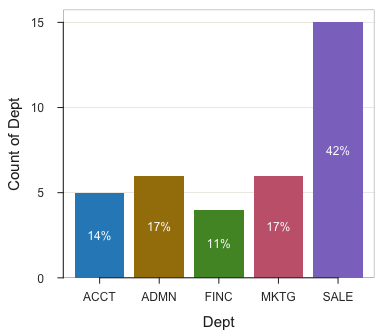Bar chart of tablulated counts of employees in each department.

## >>> Suggestions
## BarChart(Dept, horiz=TRUE)  # horizontal bar chart
## BarChart(Dept, fill="greens")  # sequential green bars
## PieChart(Dept)  # doughnut (ring) chart
## Plot(Dept)  # bubble plot
## Plot(Dept, stat="count")  # lollipop plot
##
##
## --- Dept ---
##
##
## Missing Values of Dept: 1
##
##
##                 ACCT   ADMN   FINC   MKTG   SALE    Total
## Frequencies:       5      6      4      6     15       36
## Proportions:   0.139  0.167  0.111  0.167  0.417    1.000
##
##
## Chi-squared test of null hypothesis of equal probabilities
##   Chisq = 10.944, df = 4, p-value = 0.027

The default color theme, "colors", fills the bars in the bar chart with with different hues. See more explanation of this and related color palettes more in the vignette Customize.

The BarChart() function also labels each bar with the associated numerical value. The function also provides the corresponding frequency distribution, the table that lists the count of each category, from which the bar chart is constructed.

We do not need to see this output to the R console repeated again for different bar charts of the same data, so turn off for now with the parameter quiet set to TRUE. Can set this option for each call to BarChart(), or can set for subsequent analyses with the style() function.

style(quiet=TRUE)

### Custom Colors

Specify a single fill color with the fill parameter, the edge color of the bars with color, and a horizontal bar chart with base R parameter horiz. Turn off console output. Turn off the displayed value on each bar with the parameter values set to off.

BarChart(Dept, fill="darkred", horiz=TRUE, values="off")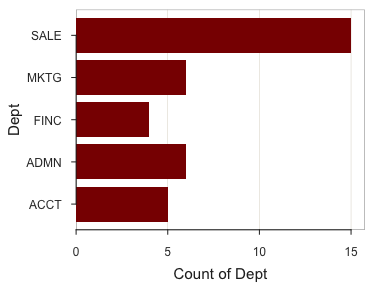Use the theme parameter to change the entire color theme: “colors”, “lightbronze”, “dodgerblue”, “darkred”, “gray”, “gold”, “darkgreen”, “blue”, “red”, “rose”, “green”, “purple”, “sienna”, “brown”, “orange”, “white”, and “light”. In this example, changing the full theme accomplishes th same as changing the fill color.

BarChart(Dept, theme="gray")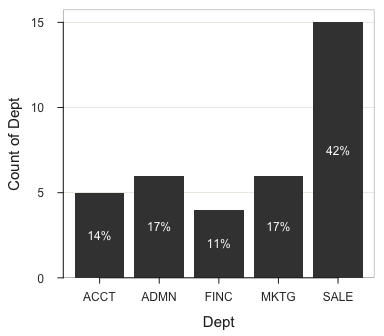Or, can use style() to change the theme for subsequent visualizations as well. See the Customize vignette.

Dept is not an ordinal variable (i.e., with ordered values), but to illustrate, can choose many different sequential palettes from getColors(): “reds”, “rusts”, “browns”, “olives”, “greens”, “emeralds”, “turquoises”, “aquas”, “blues”, “purples”, “violets”, “magentas”, and “grays”.

BarChart(Dept, fill="reds")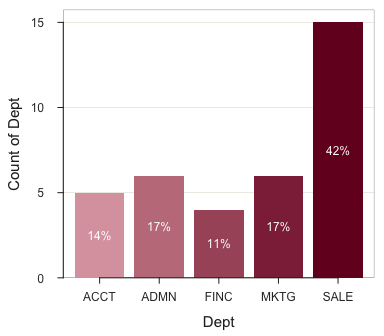### Long Value Labels

Rotate and offset the axis labels with rotate_x and offset parameters. Do a descending sort of the categories by frequencies with the sort parameter.

BarChart(Dept, rotate_x=45, offset=1, sort="-")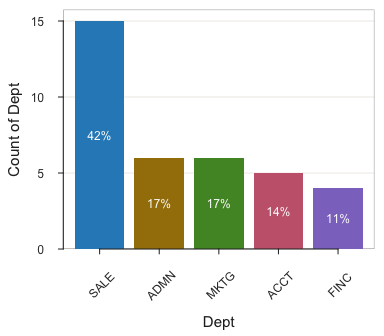Instead of setting the value of the interior color of the bars with the fill parameter, map the value of tabulated count to bar fill. With mapping, the color of the bars reflects the bar height. The higher the bar, the darker the color.

BarChart(Dept, fill=(count))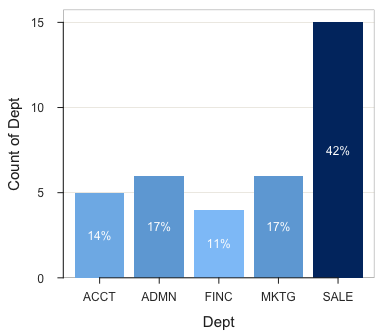### Specify the Numerical Variable

One possibility is to have the values of the $$x$$ and $$y$$ variables, such as in a table, and want to create the bar chart directly from the table. To do so, enter the paired data values into a data file such as with Excel, and then read into R with Read() When calling BarChart(), specify first the categorical $$x$$ variable and then the numerical $$y$$ variable. The general syntax follows.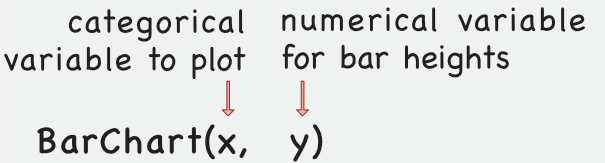Can also do a statistical transformation of $$y$$. Set the bars proportional to the height of the corresponding mean deviations of $$y$$ with the stat parameter. Possible values of stat: "sum",“mean”,“sd”,“dev”,“min”,“median”, and“max”. The“dev” value displays the mean deviations to further facilitate a comparison among levels.

Here the $$x$$-variable is Dept and $$y$$-variable is Salary. Display bars for values of dev <= 0 in a different color than values above with the fill_split parameter set at 0. Do an ascending sort with the sort parameter set at "+".

BarChart(Dept, Salary, stat="dev", sort="+", fill_split=0)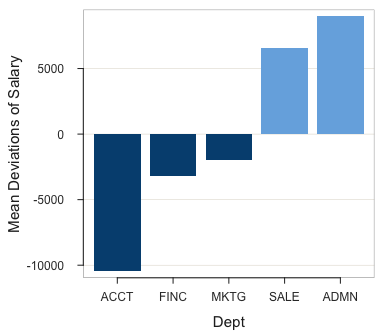### Annotation

Annotate a plot with the add parameter. To add a rectangle use the "rect" value of add. Here set the rectangle around the message centered at <3,10>. To specify a rectangle requires two corners of the rectangle, <x1,y1> and <x2,y2>. To specify text requires just a single coordinate, <x1,y1>. Because with the add parameter, the message follows the specification of "rect", the coordinates of the text message follows the coordinates for the rectangle.

First lighten the fill color of the annotation with the add_fill parameter for the style() function.

style(add_fill="aliceblue")
BarChart(Dept, add=c("rect", "Employees by\nDepartment"),
x1=c(1.75,3), y1=c(11, 10), x2=4.25, y2=9)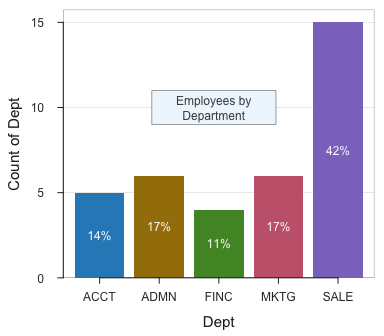## Pie Chart

An alternative to the bar chart for a single categorical variable is the pie chart.

Pie Chart: Relate each level of a categorical variable to the area of a circle (pie) scaled according to the value of an associated numerical variable.

Here the presented version of a pie chart is the doughnut or ring chart.

PieChart(Dept)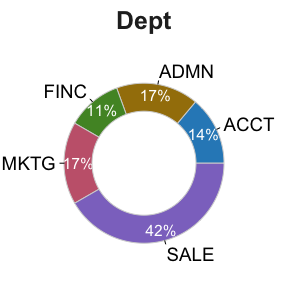The doughnut or ring chart appears easier to read than a standard bar chart. But the lessR function PieChart() also can create the “old-fashioned” pie chart by setting the value of hole to 0. We have seen the summary statistics several times now, so turn off the output to the R console here with the quiet parameter.

PieChart(Dept, hole=0, quiet=TRUE)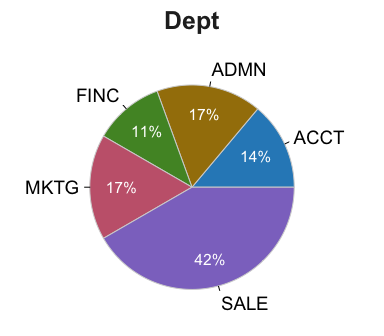Standard pie chart of variable Dept in the d data frame.

Set the size of the hole in the doughnut or ring chart with the parameter hole, which specifies the proportion of the pie occupied by the hole. The default hole size is 0.65. Set that value to 0 to close the hole.

## Bar Chart of Two Variables

### Standard Bar Charts

Specify the second categorical variable with the by parameter. Generally need to specify the by parameter by name. The general syntax follows.The example plots Dept with the percentage of Gender divided in each bar.

BarChart(Dept, by=Gender)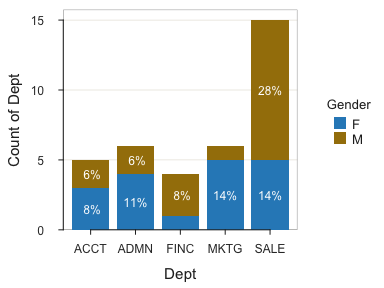The stacked version is default, but the values of the second categorical variable can also be represented with bars, more helpful to compare the values with each other.

BarChart(Dept, by=Gender, beside=TRUE)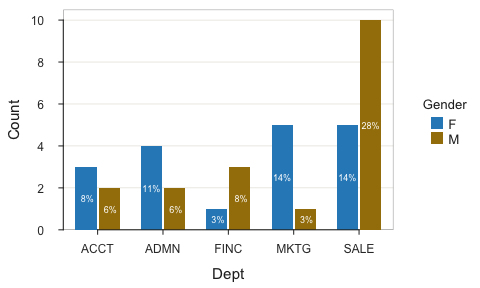### Trellis Plots

Can also do a Trellis chart with the by1 parameter.

BarChart(Dept, by1=Gender)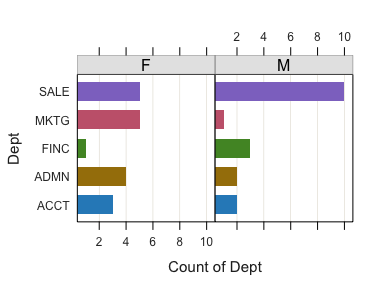Or, stack the charts vertically by specifying one column with the n_col parameter. Turn off text output to the console with the quiet parameter set to TRUE.

BarChart(Dept, by1=Gender, n_col=1, quiet=TRUE)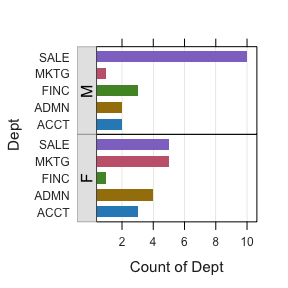### 100% Stacked Bar Chart

Obtain the 100% stacked version with the stack100 parameter. This visualization is most useful for comparing levels of the by variable across levels of the x variable, here Dept, when the frequencies in each level of the x variable differ. The comparisons are done with the percentage in each category instead of the count.

BarChart(Dept, by=Gender, stack100=TRUE)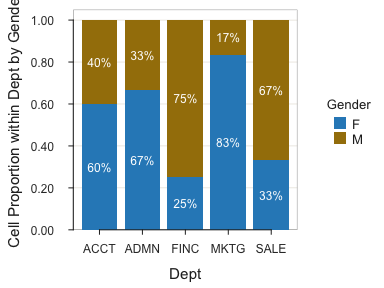### Long Value Labels

Long value labels on the horizontal axis are also addressed by moving to a new line whenever a space is encountered in the label. Here read responses to the Mach IV Machiavellianism scale where each item is scored from 0 to 5.

d <- rd("Mach4", quiet=TRUE)

Also read variable labels into the l data frame, which are then used to automatically label the output, both the visualization and text output to the console.

l <- rd("Mach4_lbl", quiet=TRUE)

Convert the specified four Mach items to factors with the lessR function factors(). A response of 0 is a Strongly Disagree, etc.

LikertCats <- c("Strongly Disagree", "Disagree", "Slightly Disagree",
"Slightly Agree", "Agree", "Strongly Agree")
d <- factors(c(m06,m07,m09,m10), levels=0:5, labels=LikertCats, ordered=TRUE)

Because the factors are defined as ordered in the factors() function, the colors are plotted in a sequential scale, from light to dark. Because output to the console has been turned off in general, turn back on just for this analysis because of new data.

BarChart(m06, by=m07, quiet=FALSE)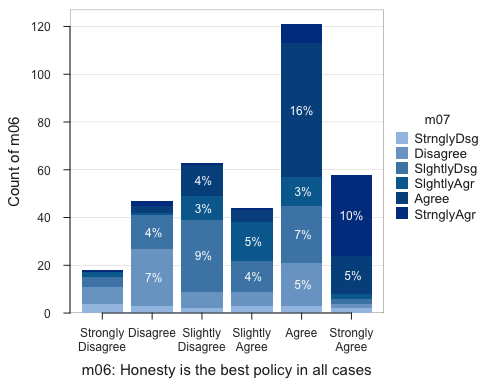## >>> Suggestions
## Plot(m06, m07)  # bubble plot
## BarChart(m06, by=m07, horiz=TRUE)  # horizontal bar chart
## BarChart(m06, fill="steelblue")  # steelblue bars
##
##
## m06: Honesty is the best policy in all cases
##   - by levels of -
## m07: There is no excuse for lying to someone else
##
## Joint and Marginal Frequencies
## ------------------------------
##
##                    m06
## m07                 Strongly Disagree Disagree Slightly Disagree Slightly Agree Agree Strongly Agree Sum
##   Strongly Disagree                 4        3                 2              3     3              2  17
##   Disagree                          7       24                 7              6    18              2  64
##   Slightly Disagree                 4       14                30             13    24              2  87
##   Slightly Agree                    2        1                10             16    12              2  43
##   Agree                             0        3                13              5    56             16  93
##   Strongly Agree                    1        2                 1              1     8             34  47
##   Sum                              18       47                63             44   121             58 351
##
##
## Cramer's V: 0.380
##
## Chi-square Test:  Chisq = 253.103, df = 25, p-value = 0.000
## >>> Low cell expected frequencies, chi-squared approximation may not be accurate

## Full Manual

Use the base R help() function to view the full manual for BarChart(). Simply enter a question mark followed by the name of the function.

?BarChart`

## More

More on Bar Charts and other visualizations from lessR and other packages such as ggplot2 at:

Gerbing, D., R Visualizations: Derive Meaning from Data, CRC Press, May, 2020, ISBN 978-1138599635.